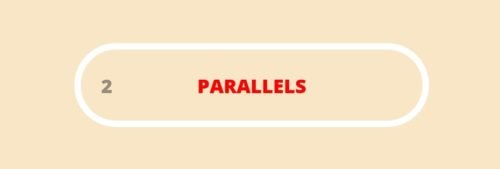# Pythagorea Parallels Level 2.16 Solution/AnswerPythagorea Parallels Level 2.16 New Version Game Answers,  detailed solutions, Tips, and Walkthrough. Scroll below to find answer to this level.

Pythagorea is android/iOS app developed by Horis International Limited. Solutions hints and answers to pythagorea are available in this post scroll down to find solutions to all the levels.

This game is mostly focused on geometric puzzles and construction. The workspace is divided into grids to draw lines. You should know all the basic Math operations. All lines and shapes are drawn on a grid whose cells are squares. Most of the game levels can be answered using natural intuition and by some basic laws of geometry.Pythagorea parallels 2.16: Construct a line through the point A that is parallel to the given line.If you have any doubt regarding Pythagorea answers given here you can watch video below or you can comment on this post-

### 16 thoughts on “Pythagorea Level 2.16 Answer Solution”

•September 8, 2019 at 7:44 am

Not-so-elegant solution using x-y equations:
1. Use (0,0) at the bottom left as the reference point for representing the entire 6 x 6 grid as an x,y coordinate plane. Given that as what is established, the given line would be represented by
y = (3/5)x.
2. Since point A is at (2,4), find y at the given line when x = 2, which is 6/5. Find the difference in y-coordinates between point A and (2, 6/5): 4 – 6/5 = 20/5 – 6/5 = 14/5. This gives you the y-translation to find the second equation that intersects point A: y = (3/5)x + 14/5
3. Pick an (x, y) value within the visible 6 x 6 grid for your newly found equation. I’m going to choose x = 4, which will give you y = (26/5).

4.
The not-so-elegant part:
Find and draw a line segment that will hit (4, 26/5). This line segment will have to have a slope that will go a fifth of a y-unit up whenever the x-value traverses 1 unit left or right. The line segment will be up to your choosing. I chose the one between (5,5) and (0,6).

5. Draw the line between point A and (4, 26/5).

•July 23, 2019 at 8:24 am

Perfect! Thanks a lot.
From the original triangle:
5/3=3/x then x=9/5

5/4=1/y then y=4/5

So, y+1=4/5 + 5/5=x
Very smart! Congratulations.

•September 20, 2018 at 6:50 am

You might have had to use math. Just saying.

•August 3, 2018 at 1:05 pm

Think of it like this: the slope is 3/5 so you can expect the y value at x=5 (if the origin is the bottom left) to be 4 + (3/5)*3 = 5 + 4/5 = 6 – 1/5. In order to make a point at (5, 6 – 1/5), construct a line with a slope of 1/5 so that for each x you travel left, the value decreases by 1/5. As long as this line intersects (6, 6), it will intersect (5, 6 – 1/5) also. This approach also works if you make the endpoints of the secondary line at (0,4) and (5,5). The paralell line will intersect the secondary line at (3, 4 + 3/5).

•June 30, 2018 at 8:12 pm

Every 3 along, the line goes up 1.8 (gradient 3/5). The construction line allows the square grid to be split into fifths vertically. So from A, 3 along and 1.8 up is where the construction point has gone, keeping gradient same as original. Ok?

•May 22, 2018 at 4:29 pm

Just because your “solution” is correct doesn’t mean you used math to get it. Your choice of line to get the second point on the parallel line seems chosen at random.

•June 30, 2018 at 3:07 pm

The original line goes along 3 and up 9/5 (gradient of 3/5). The construction line allows the vertical distances to be split into fifths, so the constructed point is 3 along and 9/5 up from point A, allowing lines to be parallel.

•April 28, 2018 at 2:34 pm

I too don’t see any math involved in this solution.

•June 30, 2018 at 3:01 pm

Every 3 along, the line goes up 1.8 (gradient 5/3). The construction line allows the square grid to be split into fifths vertically. So from A, 3 along and 1.8 up is where the construction point has gone, keeping gradient same as original. Ok?

•April 11, 2018 at 3:40 pm

Went to link. Looked at the drawing. Line AF does not have a 5/3 slope. Line BC does. How can they be parallel?

•April 10, 2018 at 5:38 pm

2.16 “solution” is incorrect. It is not based on any math, and you can see that the vertcal distance between point A and the line and another point on the new line to the original line is different.

•April 11, 2018 at 1:09 pm

Go to this link to find out why it is correct, I tried online simulator to check.

•April 11, 2018 at 3:56 pm

Yep, I don’t get how the provided solution is correct. AF line has a slope of -8/5 and the CB line has a slope of -5/3 They will intersect.

•April 11, 2018 at 5:37 pm
•